Technical Information Site of Power Supply Design

• Basic Knowledge
• DC/DC
• Examination of Losses
• Matters to Consider When Studying Miniaturization by Raising the Switching Frequency

2019.04.25 DC/DC

# Matters to Consider When Studying Miniaturization by Raising the Switching Frequency

Examination of Losses

In the previous article, loss factors under various conditions were explained. From this article, in order to address specifications required of applications, we explain loss factors that should be noted when studying circuit operation and the like, as well as countermeasures.

Matters to Consider When Studying Application Miniaturization by Raising the Switching Frequency

By raising the switching frequency of a switching DC/DC converter circuit, the component values of external inductors and capacitors can be decreased. As a result, inductors and capacitors in smaller shapes and with smaller packages can be used, the mounting area required by the circuit is reduced, and the equipment size can be made more compact. This is an approach that is frequently studied when designing compact portable devices and equipment.

As explained in the previous article, there are three loss factors that are affected by the switching frequency fSW: ① gate charge losses, ② switching losses, and ③ dead time losses.

We calculate the actual amount by which these losses increase when the switching frequency is raised. The conditions are the conditions used on the right side in”Example of Power Loss Calculation for a Power Supply IC”. The switching frequency is raised from 0.1 MHz to 2 MHz.

Below are the formulae used and actual calculated results. Gate charge losses are summarized for the H-side and L-side.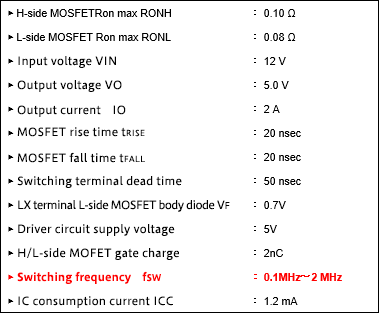Loss factors that increase as the frequency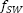rises

①Gate charge loss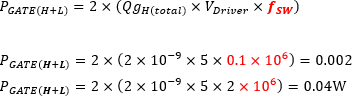②Switching loss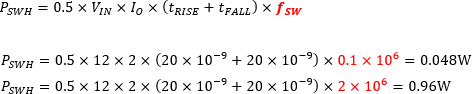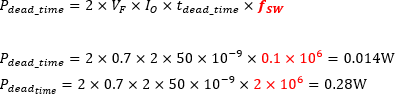The switching frequency fSW has increased 20-fold, from 0.1 MHz to 2 MHz, and so as is clear from the equations, all loss powers are simply increased 20-fold. However, when considering the proportions of the losses in the total loss power, the switching loss ② and the dead time loss ③ are dominant. Below is a graphical representation of the respective losses as functions of the switching frequency.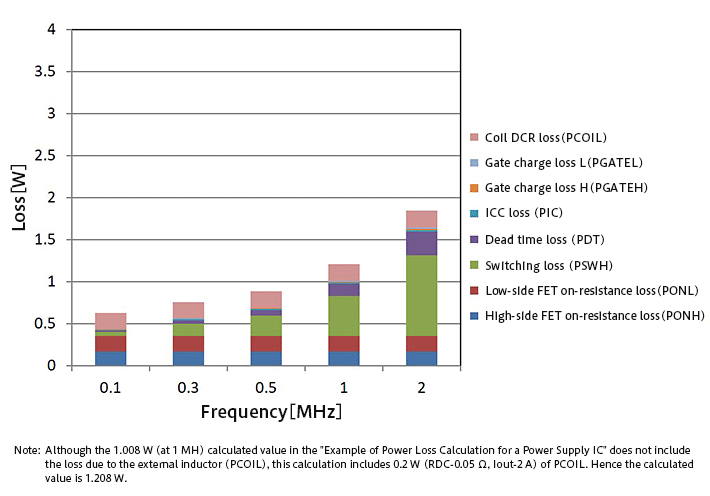To indicate the overall loss using specific numeric values, when the switching frequency is 0.1 MHz, the loss is 0.632 W, at 1 MHz the loss is 1.208 W, and at 2 MHz the loss is 1.848 W. Thus the loss clearly increases as the switching frequency rises.

Upon calculating the efficiency, because the output power is 10 W (5 V/2 A) and the input power is the output power plus the loss power, at 0.1 MHz the efficiency is 94.1%, at 1 MHz it is approximately 89.2%, and at 2 MHz it is 84.4%, so that in a change from 1 MHz to 2 MHz that is likely to occur, the efficiency drops by as much as 4.8%.

Examination and Countermeasures

By raising the switching frequency, smaller external inductors and capacitors can be used, and so power supplies and applications can be made more compact. However, by raising the switching frequency, the switching loss and dead time loss are increased, and efficiency drops. That is, one could say that there is a trade-off between compactness through higher switching frequencies and increases in losses (declines in efficiency).

As countermeasures, based on the demands of the application, the switching frequency should be set on the basis of the losses that can be allowed (efficiency issues) and the equipment size. If size is given top priority, the highest switching frequency possible should be used; if efficiency is paramount, the lowest switching frequency should be chosen. In many cases, it will be necessary to compromise with a balance between size and efficiency.

#### Key Points:

・By increasing the switching frequency, the power supply and application can be made smaller, but losses increase and efficiency suffers.

・Among increasing losses, switching losses and dead time losses are dominant.

・There is a trade-off between miniaturization through higher switching frequencies and increases in losses (reduced efficiency).

・In many cases, a balance must be sought between size and efficiency.